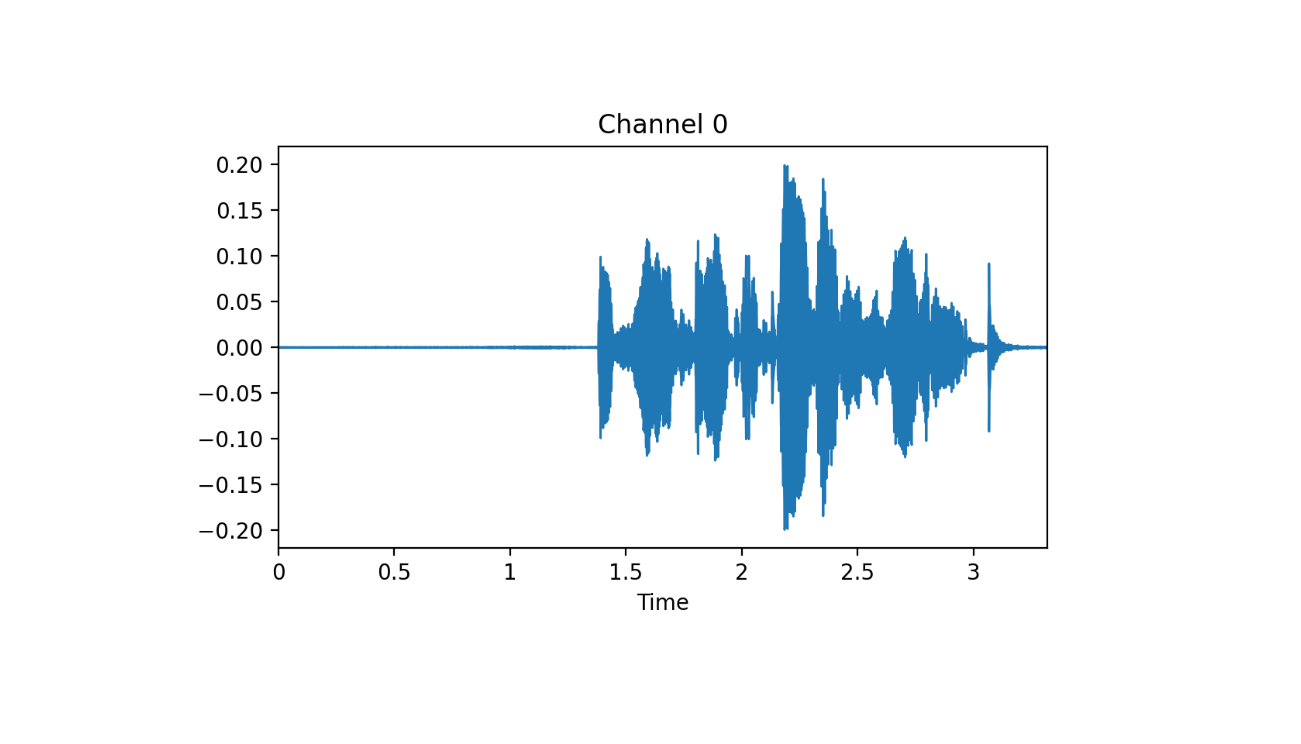# Audio Classification

## Intro

The fastai library simplifies training fast and accurate neural nets using modern best practices. See the fastai website to get started. The library is based on research into deep learning best practices undertaken at fast.ai, and includes “out of the box” support for vision, text, tabular, and collab (collaborative filtering) models.

## FastAudio

For now, the library can be installed via git. However, later it will be available on pypi:

reticulate::py_install('git+https://github.com/fastaudio/fastaudio.git', pip = TRUE)

## Dataset

Grab data:

URLs_SPEAKERS10()
path_dig = 'SPEAKERS10'

See audio extensions:

audio_extensions()[1:6]
# ".aif"  ".aifc" ".aiff" ".au"   ".m3u"  ".mp2" 

fnames = get_files(path_dig, extensions = audio_extensions())
# (#3842) [Path('SPEAKERS10/f0004_us_f0004_00414.wav')...]

## Visualize

Read audio data and visualize a tensor:

at = AudioTensor_create(fnames)
at; at$shape at %>% show() %>% plot(dpi = 200)## Preparing the dataset fastaudio has a AudioConfig class which allows us to prepare different settings for our dataset. Currently it has: • BasicMelSpectrogram • BasicMFCC • BasicSpectrogram • Voice Voice module is the most suitable because it contains human voices. cfg = Voice() cfg$f_max; cfg$sample_rate # 8000 # frequency range # 16000 # the sampling rate Turn data into spectrogram and crop signal: aud2spec = AudioToSpec_from_cfg(cfg) crop1s = ResizeSignal(1000) Create a pipeline and see the result: pipe = Pipeline(list(AudioTensor_create, crop1s, aud2spec)) pipe(fnames) %>% show() %>% plot(dpi = 200) ## Dataloader As usual, prepare a datalaoder: item_tfms = list(ResizeSignal(1000), aud2spec) get_y = function(x) substring(x$name,1,1)

aud_digit = DataBlock(blocks = list(AudioBlock(), CategoryBlock()),
get_items = get_audio_files,
splitter = RandomSplitter(),
item_tfms = item_tfms,
get_y = get_y)

dls = aud_digit %>% dataloaders(source = path_dig, bs = 64)

dls %>% show_batch(figsize = c(15, 8.5), nrows = 3, ncols = 3, max_n = 9, dpi = 180)

## Pretrained model

We will use a pretrained ResNet model. However, the channel number and weight dimension have to be changed:

torch = torch()
nn = nn()

alter_learner = function(learn, channels = 1L) {
learn$model[['in_channels']] %f% channels learn$model[['weight']] %f% torch$nn$parameter$Parameter( (learn$model$weight %>% narrow('[:,1,:,:]'))$unsqueeze(1L)
)
}

learn = Learner(dls, xresnet18(pretrained = FALSE), nn$CrossEntropyLoss(), metrics=accuracy) nnchannels = dls %>% one_batch() %>% .[] %>% .$shape %>% .
alter_learner(learn, nnchannels)

Find lr:

lrs = learn %>% lr_find()
#SuggestedLRs(lr_min=0.03019951581954956, lr_steep=0.0030199517495930195)

## Conclusion

And fit:

learn %>% fit_one_cycle(10, 1e-3)
epoch     train_loss  valid_loss  accuracy  time
0         5.494162    3.295561    0.632812  00:06
1         1.962470    0.236809    0.877604  00:06
2         0.801965    0.174774    0.917969  00:06
3         0.391742    0.208425    0.881510  00:06
4         0.243276    0.149436    0.914062  00:06
5         0.174708    0.134832    0.929688  00:07
6         0.142626    0.127814    0.910156  00:06
7         0.131042    0.120308    0.924479  00:07
8         0.121679    0.126913    0.919271  00:06
9         0.118215    0.114659    0.924479  00:06